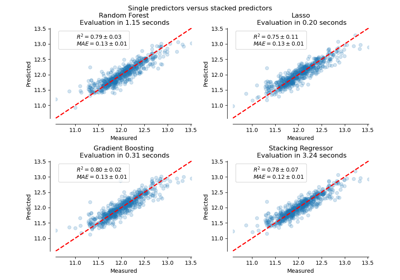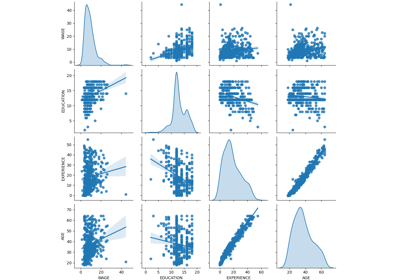sklearn.model_selection.cross_validate¶

sklearn.model_selection.cross_validate(estimator, X, y=None, *, groups=None, scoring=None, cv=None, n_jobs=None, verbose=0, fit_params=None, pre_dispatch='2*n_jobs', return_train_score=False, return_estimator=False, error_score=nan)[source]

Evaluate metric(s) by cross-validation and also record fit/score times.

Read more in the User Guide.

Parameters
estimatorestimator object implementing ‘fit’

The object to use to fit the data.

Xarray-like of shape (n_samples, n_features)

The data to fit. Can be for example a list, or an array.

yarray-like of shape (n_samples,) or (n_samples, n_outputs), default=None

The target variable to try to predict in the case of supervised learning.

groupsarray-like of shape (n_samples,), default=None

Group labels for the samples used while splitting the dataset into train/test set. Only used in conjunction with a “Group” cv instance (e.g., GroupKFold).

scoringstr, callable, list/tuple, or dict, default=None

A single str (see The scoring parameter: defining model evaluation rules) or a callable (see Defining your scoring strategy from metric functions) to evaluate the predictions on the test set.

For evaluating multiple metrics, either give a list of (unique) strings or a dict with names as keys and callables as values.

NOTE that when using custom scorers, each scorer should return a single value. Metric functions returning a list/array of values can be wrapped into multiple scorers that return one value each.

See Specifying multiple metrics for evaluation for an example.

If None, the estimator’s score method is used.

cvint, cross-validation generator or an iterable, default=None

Determines the cross-validation splitting strategy. Possible inputs for cv are:

• None, to use the default 5-fold cross validation,

• int, to specify the number of folds in a (Stratified)KFold,

• An iterable yielding (train, test) splits as arrays of indices.

For int/None inputs, if the estimator is a classifier and y is either binary or multiclass, StratifiedKFold is used. In all other cases, KFold is used.

Refer User Guide for the various cross-validation strategies that can be used here.

Changed in version 0.22: cv default value if None changed from 3-fold to 5-fold.

n_jobsint, default=None

The number of CPUs to use to do the computation. None means 1 unless in a joblib.parallel_backend context. -1 means using all processors. See Glossary for more details.

verboseint, default=0

The verbosity level.

fit_paramsdict, default=None

Parameters to pass to the fit method of the estimator.

pre_dispatchint or str, default=’2*n_jobs’

Controls the number of jobs that get dispatched during parallel execution. Reducing this number can be useful to avoid an explosion of memory consumption when more jobs get dispatched than CPUs can process. This parameter can be:

• None, in which case all the jobs are immediately created and spawned. Use this for lightweight and fast-running jobs, to avoid delays due to on-demand spawning of the jobs

• An int, giving the exact number of total jobs that are spawned

• A str, giving an expression as a function of n_jobs, as in ‘2*n_jobs’

return_train_scorebool, default=False

Whether to include train scores. Computing training scores is used to get insights on how different parameter settings impact the overfitting/underfitting trade-off. However computing the scores on the training set can be computationally expensive and is not strictly required to select the parameters that yield the best generalization performance.

New in version 0.19.

Changed in version 0.21: Default value was changed from True to False

return_estimatorbool, default=False

Whether to return the estimators fitted on each split.

New in version 0.20.

error_score‘raise’ or numeric

Value to assign to the score if an error occurs in estimator fitting. If set to ‘raise’, the error is raised. If a numeric value is given, FitFailedWarning is raised. This parameter does not affect the refit step, which will always raise the error.

New in version 0.20.

Returns
scoresdict of float arrays of shape (n_splits,)

Array of scores of the estimator for each run of the cross validation.

A dict of arrays containing the score/time arrays for each scorer is returned. The possible keys for this dict are:

test_score

The score array for test scores on each cv split. Suffix _score in test_score changes to a specific metric like test_r2 or test_auc if there are multiple scoring metrics in the scoring parameter.

train_score

The score array for train scores on each cv split. Suffix _score in train_score changes to a specific metric like train_r2 or train_auc if there are multiple scoring metrics in the scoring parameter. This is available only if return_train_score parameter is True.

fit_time

The time for fitting the estimator on the train set for each cv split.

score_time

The time for scoring the estimator on the test set for each cv split. (Note time for scoring on the train set is not included even if return_train_score is set to True

estimator

The estimator objects for each cv split. This is available only if return_estimator parameter is set to True.

sklearn.model_selection.cross_val_score

Run cross-validation for single metric evaluation.

sklearn.model_selection.cross_val_predict

Get predictions from each split of cross-validation for diagnostic purposes.

sklearn.metrics.make_scorer

Make a scorer from a performance metric or loss function.

Examples

>>> from sklearn import datasets, linear_model
>>> from sklearn.model_selection import cross_validate
>>> from sklearn.metrics import make_scorer
>>> from sklearn.metrics import confusion_matrix
>>> from sklearn.svm import LinearSVC
>>> X = diabetes.data[:150]
>>> y = diabetes.target[:150]
>>> lasso = linear_model.Lasso()

Single metric evaluation using cross_validate

>>> cv_results = cross_validate(lasso, X, y, cv=3)
>>> sorted(cv_results.keys())
['fit_time', 'score_time', 'test_score']
>>> cv_results['test_score']
array([0.33150734, 0.08022311, 0.03531764])